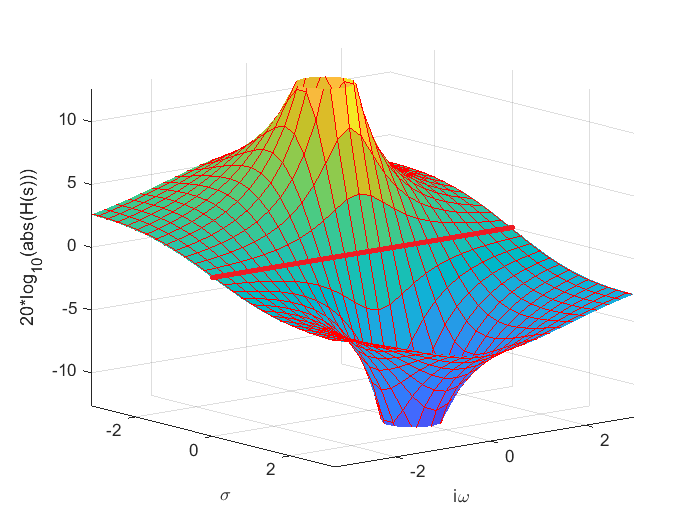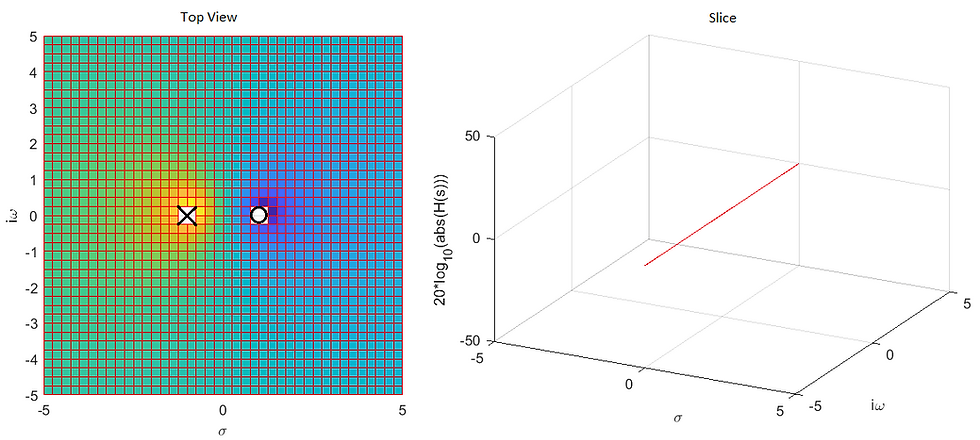top of page

# #016: Poles and zeros, the real heroes

With an engineering degree in electronics, I have been comfortable working with transfer functions and bode plots for years. Even though I later went into physics and simulations, it has been extremely helpful to have this knowledge, e.g. when doing rather complex lumped parameter analogy circuits of vibroacoustic applications. In today's blog post we will have a look at how the so-called poles and zeros tie together with transfer functions in a graphical manner that is not often shown in the electronics books.

As mentioned in a previous blog post, I was very fascinated by active crossovers some years back. I managed to do my first journal paper on this very topic, while looking for a job after finishing my master's thesis, and spent a lot of time reading books and visiting e.g. www.linkwitzlab.com (Incidentally, Linkwitz later cited my paper http://www.linkwitzlab.com/crossovers.htm) and other sites on the topic. When dealing with passive and active filters you get very used to looking at so-called transfer functions. An examples is shown below; a second order high-pass filter with unity output at high frequencies:

H is the transfer funtion, which tells all you need to need to know about the system properties, both in time and frequency domain. The variable s comes from the Laplace transformation. This transformation allows us to convert complicated differential equations into polynomials that can be dealt with algebraically, but all we will say about that for now is that s is complex and equal to a real part and an imaginary part as

where we note that even though it is practice in engineering to use "j" to indicate the imaginary part, I instead stick with the mathematics convention "i". Since s is complex and H is a function of s, it follows that H is a complex function with a complex argument. More on that later... An general way of writing a transfer function is in the form of poles and zeros with the polynomial fraction written as factorized subtractions:

Here z indicates a zero, and p a pole, and the factor k is scales the overall amplification. When s equals any z, the complex transfer function equals zero, and when s equals any pole the expression goes to infinity. We can write our specific second-order high-pass filter in a factorized form as

We note that we have two zeros at s=0, and two poles, that may or may not be complex, depending on Q. If we as an example set the characteristic angular frequency to 1, and the quality factor Q also to 1, we find that the poles are (-0.5+i 0.5√(3)) and (-0.5-i 0.5√(3)). Knowing the zeros and poles, and thus the characteristic frequency and quality factor, allows us to plot both a pole-zero diagram and the magnitude of the transfer function:Now what I really want to convey with this blog post, is that the plots above are actually one and the same, just seen from different perspectives! The first time I saw this visualized, was in an excellent DSP book, and it blew my mind. I couldn't believe that this had never dawned on me, as I had actually finished my MSc at the time. Let me illustrate with a simpler example first, a first-order all-pass transfer function:

The zero and the pole are seen directly from the transfer function. As mentioned above, H is a complex function with a complex argument, so it needs two plots to shown properly, either as magnitude and phase as function of s in a complex plane, or as real or imaginary values of H as a function of s in a complex plane. Let's have look at the amplitude, plotted logarithmically, for a characteristic angular frequency of 1:So at the zero (s=1), the response goes to zero, and hence the logarithm goes to minus infinity. At the pole (s=-1) the response goes to infinity. How does that relate to the transfer function? Well, let's change the plot at little by adding grid lines to H(s) and changing the viewing angle:Note how there in the entire landscape is only one straight path, highlighted in red, which has a constant value along that path; namely along sigma=0. The value found along this path is 0 dB, i.e. H(s)=1, for this particular case, and this is exactly the magnitude of our all-pass transfer function! This is due to the fact that at this line, the distance to the pole and the distance to the zero is equal, and this can be expressed more generally when we have multiple poles and zeros, but we won't get into that right now. So we see that the pole-zero plot and the transfer function are really interconnected and can be seen as top view and a slice, respectively, of the above plot:So the peaks and valleys created by the zeros and poles, make up the transfer function at sigma=0. This value is the only value that makes physical sense, but still the placement of the zeros and poles completely dictate what goes on along the frequency axis. Also, note that we usually only consider positive frequency values, so the above slice could be cut further.

We can now go back to our second-order high-pass filter example and apply the same technique, and see how the H(s) plot, the pole-zero plot, and the transfer function all change, as we step through several Q-values for a given characteristic angular frequency of 1:Again, these plots are the same, just from different perspectives.

By the way, I used MATLAB for all plots above, but if you want to play around with some of these transfer funtion, and only have an electronics program, you can still have a look at the transfer funtions. For example, the all-pass functionality can realized with the following implementation:The transfer function for this circuit is

and we see clearly that there is a pole at s=-1/RC, and a zero at s=1/RC. We have assumed that R1 and the op-amp have no tolerances. However, remember from my previous post on sensitivity analysis, that all circuits have tolerances in all their components, so the actual transfer function may differ from the theoretical one. We can visualize this via the pole-zero plot, but I will leave that as an exercise to the reader. Due to the nature of the all-pass setup, any tolerances in R and C will move the pole and the zero equally, so that that the all-pass functionality is retained, but the phase response will differ as the characteristic frequency changes. You can also try out different filter configurations here.

And that is it, I hope you learned something new, or at least got a refresher course in pole-zero analysis.

Happy Easter!

Tags:

bottom of page